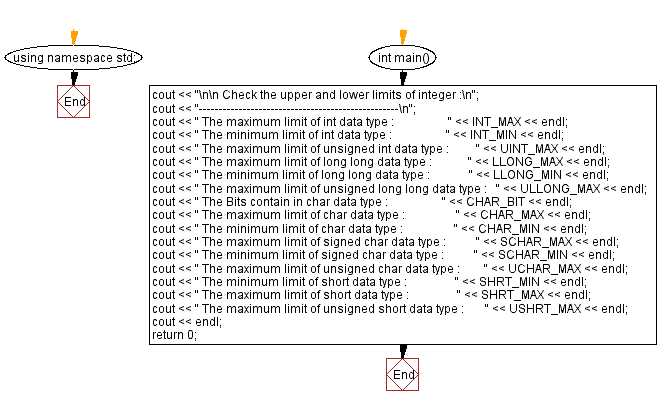﻿ C++ Exercises: Check the upper and lower limits of integer - w3resource

# C++ Exercises: Check the upper and lower limits of integer

## C++ Basic: Exercise-5 with Solution

Write a in C++ program to check the upper and lower limits of integers.

Sample Solution:

C++ Code :

``````#include <iostream>
#include <climits>   // integer limits in header file
using namespace std;

int main()
{
cout << "\n\n Check the upper and lower limits of integer :\n";
cout << "--------------------------------------------------\n";
cout << " The maximum limit of int data type :                  " << INT_MAX << endl;
cout << " The minimum limit of int data type :                  " << INT_MIN << endl;
cout << " The maximum limit of unsigned int data type :         " << UINT_MAX << endl;
cout << " The maximum limit of long long data type :            " << LLONG_MAX << endl;
cout << " The minimum limit of long long data type :             " << LLONG_MIN << endl;
cout << " The maximum limit of unsigned long long data type :   " << ULLONG_MAX << endl;
cout << " The Bits contain in char data type :                  " << CHAR_BIT << endl;
cout << " The maximum limit of char data type :                 " << CHAR_MAX << endl;
cout << " The minimum limit of char data type :                 " << CHAR_MIN << endl;
cout << " The maximum limit of signed char data type :          " << SCHAR_MAX << endl;
cout << " The minimum limit of signed char data type :          " << SCHAR_MIN << endl;
cout << " The maximum limit of unsigned char data type :        " << UCHAR_MAX << endl;
cout << " The minimum limit of short data type :                " << SHRT_MIN << endl;
cout << " The maximum limit of short data type :                " << SHRT_MAX << endl;
cout << " The maximum limit of unsigned short data type :       " << USHRT_MAX << endl;
cout << endl;
return 0;
}
``````

Sample Output:

``` Check the upper and lower limits of integer :
--------------------------------------------------
The maximum limit of int data type :                  2147483647
The minimum limit of int data type :                  -2147483648
The maximum limit of unsigned int data type :         4294967295
The maximum limit of long long data type :            9223372036854775807
The minimum limit of long long data type :             -9223372036854775808
The maximum limit of unsigned long long data type :   18446744073709551615
The Bits contain in char data type :                  8
The maximum limit of char data type :                 127
The minimum limit of char data type :                 -128
The maximum limit of signed char data type :          127
The minimum limit of signed char data type :          -128
The maximum limit of unsigned char data type :        255
The minimum limit of short data type :                -32768
The maximum limit of short data type :                32767
The maximum limit of unsigned short data type :       65535
```

Flowchart:C++ Code Editor:

What is the difficulty level of this exercise?

﻿

## C++ Programming: Tips of the Day

Why is there no std::stou?

The most pat answer would be that the C library has no corresponding "strtou", and the C++11 string functions are all just thinly veiled wrappers around the C library functions: The std::sto* functions mirror strto*, and the std::to_string functions use sprintf.

Ref: https://bit.ly/3wtz2qA

We are closing our Disqus commenting system for some maintenanace issues. You may write to us at reach[at]yahoo[dot]com or visit us at Facebook